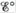Chapter 18, Problem 50E

Chapter
Section
Textbook Problem

# The amount of manganese in steel is determined by changing it to permanganate ion. The steel is first dissolved in nitric acid, producing Mn2+ ions. These ions are then oxidized to the deeply colored MnO4− ions by periodate ion (IO4−) in acid solution.a. Complete and balance an equation describing each of the above reactions.b. Calculateand ΔG° at 25°C for each reaction.

(a)

Interpretation Introduction

Interpretation: The complete and balanced given chemical reaction corresponding to the stated reaction is to be determined. The standard cell potential Eο and standard Gibbs free energy ΔGο at 2οC are to be calculated.

Concept introduction: The chemical equation is said to be balanced when the number of atoms of chemical species on the reactant side is equal to the number of chemical species on the product side. Cell potential is defined as the measure of energy per unit charge available from the redox reaction to carry out the reaction. Gibbs free energy is a thermodynamic quantity that is used to calculate the maximum work of reversible reaction performed by a system. It is equal to the difference between the enthalpy and the product of entropy at absolute temperature.

To determine: The complete and balanced given chemical reaction.

Explanation

Explanation

The balanced chemical reaction representing the dissolution of steel in nitric acid to produce Mn2+ is as follows,

3Mn(s)+8H+(aq)+2NO3-(aq)3Mn2+(aq)+2NO(g)+4H2O(l) (1)

The balanced chemical reaction representing the oxidation of Mn2+ ions into deeply colored

(b)

Interpretation Introduction

Interpretation: The complete and balanced given chemical reaction corresponding to the stated reaction is to be determined. The standard cell potential Eο and standard Gibbs free energy ΔGο at 2οC are to be calculated.

Concept introduction: The chemical equation is said to be balanced when the number of atoms of chemical species on the reactant side is equal to the number of chemical species on the product side. Cell potential is defined as the measure of energy per unit charge available from the redox reaction to carry out the reaction. Gibbs free energy is a thermodynamic quantity that is used to calculate the maximum work of reversible reaction performed by a system. It is equal to the difference between the enthalpy and the product of entropy at absolute temperature.

To determine: The standard cell potential Eο and standard Gibbs free energy ΔGο at 2οC .

### Still sussing out bartleby?

Check out a sample textbook solution.

See a sample solution

#### The Solution to Your Study Problems

Bartleby provides explanations to thousands of textbook problems written by our experts, many with advanced degrees!

Get Started